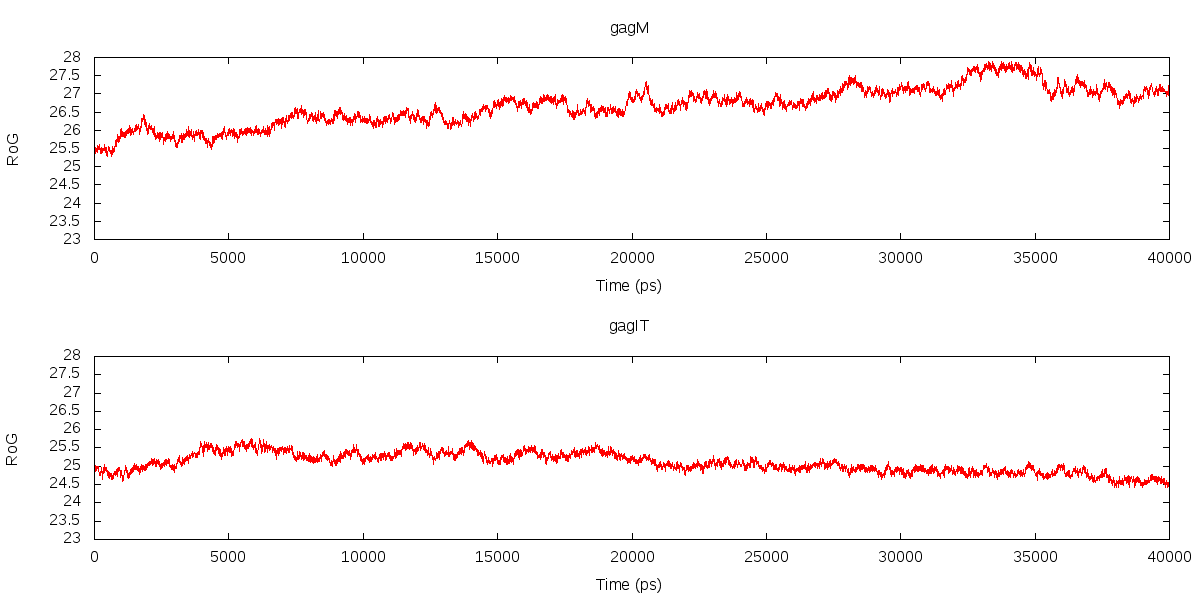# Re: [AMBER] question of Radius of Gyration

From: Chinh Su Tran To <chinh.sutranto.gmail.com>
Date: Fri, 5 Jul 2013 13:00:48 +0800

Dear Dr. Roe,

Thank you.
Btw, I ran 40 ns MD for a 500 residue molecule. I didnt set any value for
the "iwrap", so I think it was default as iwrap =0.

This is my md.in:
*#running equilibration for 10ns to give the sys time to relax*
* &cntrl*
* imin=0,*
* irest=1,*
* ntx=7,*
* ntb=2,*
* pres0=1.0,*
* ntp=1,*
* taup=2.0,*
* cut=10.0,*
* ntr=0,*
* ntc=2,*
* ntf=2,*
* tempi=300.0,*
* temp0=300.0,*
* ntt=3,*
* gamma_ln=1.0,*
* nstlim=5000000,*
* dt=0.002,*
* ntpr=2500,*
* ntwx=2500,*
* ntwr=2500*
* /*

This time I run:

And I got the result as attached. According to the plot, COULD I draw a
conclusion that the model at the bottom is more stable than the upper one
regardless their values??

Im wondering if there is any threshold to determine that the model is
stable (or reaching some reasonable compactness?).
As in my case the values are around 25+.

Regards,
Chinh

On Fri, Jul 5, 2013 at 1:36 AM, Daniel Roe <daniel.r.roe.gmail.com> wrote:

> Hi,
>
> On Thu, Jul 4, 2013 at 5:55 AM, Chinh Su Tran To
> <chinh.sutranto.gmail.com> wrote:
> > *radgyr out gag_RoG.dat mass*
>
> You're not specifying an atom mask here, so if you have ions/solvent
> calculation. Without knowing more details of your simulation I can
> only speculate, but if you were performing an explicit solvent
> simulation with no coordinate wrapping (i.e. iwrap=0) you might expect
> the total RoG of the entire system to increase over the simulation
> like that.
>
> > => Does that mean here the RadGyr is the average values of all the atoms
> in
> time
> > value, i.e. how long is the interval here!!) ?
>
> RadiusOfGyration = sqrt( SUM[ dxyz^2 * mass ] / totalMass )
>
> where the sum is over all selected atoms and dxyz^2 is the distance
> squared from each atom to the center of mass of all selected atoms.
> This calculation is performed for every coordinate frame.
>
> > Then in this case, how the MaxRad could be interpreted?
>
> MaxRad is the maximum observed distance during the radius of gyration
> calculation.
>
> -Dan
>
> --
> -------------------------
> Daniel R. Roe, PhD
> Department of Medicinal Chemistry
> University of Utah
> 30 South 2000 East, Room 201
> Salt Lake City, UT 84112-5820
> http://home.chpc.utah.edu/~cheatham/
> (801) 587-9652
> (801) 585-9119 (Fax)
>
> _______________________________________________
> AMBER mailing list
> AMBER.ambermd.org
> http://lists.ambermd.org/mailman/listinfo/amber
>

_______________________________________________
AMBER mailing list
AMBER.ambermd.org
http://lists.ambermd.org/mailman/listinfo/amber